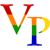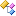﻿Vts.Modeling NamespaceVts.Modeling Namespace Virtual Photonics - VTS Library
Virtual Photonics

Classes

ClassDescriptionCalculatorToolbox
Toolbox containing general method for the forward models. Most of these are for diffusion based calculations.HankelTransform
Evaluate the Hankel transform using a digital fitler method for the quadrature points. Necessary to have a continuous function for transformation.LinearDiscreteFourierTransform
Class used to compute the discrete Fourier transform of a reflectance curve.LinearDiscreteHankelTransform
Transforms linearly discrete data via a middle Riemann sum. This method is not mathematically sound, but is a low order approximation.NumericalDerivativeExtensions